# Crank mechanism statics - free body diagrams

We devoted three tutorials to resolving by various methods the displacement, velocity and acceleration (the kinematics) of the elements in a slider and crank mechanism.  We now progress to examining forces and moments in the individual elements of the mechanism using principles of static equilibrium.

In this tutorial we develop simple free body diagrams, neglecting inertia, friction and gravitational forces, for slider and crank mechanisms with loading on power and compression strokes. For preferencel we use the term "piston" (enclosed in a cylinder) rather than "slider".

#### Free body diagrams

A free body diagram (shows forces and moments on machine elements as the first step to calculations using static equilibrium conditions (sum of forces = 0, sum of moments = 0).  In a succeeding tutorial we explain how inertia forces are included in a static force analysis using D'Alembert's principle.

#### Example 1   outward power stroke

The figure below shows the outward* power stroke of a horizontal engine producing counter-clockwise rotation of the crank arm, shown at crank angle θ = 50° . Dimensions and other parameters are identical to examples in the kinematics tutorials with piston force FP added.  The free body diagrams and calculated values of forces and moments are, of course, different for every crank angle over a complete cycle from 0°to 360°.

* For horizontal engines extremities of travel of the piston are known as inner dead centre at crank angle = 0° and outer dead centre at crank angle 180°. The corresponding terms for a vertical engine are respectively top dead centre and bottom dead centre. The notation for vertical engines is generally used for internal combustion engines regardless of their orientation.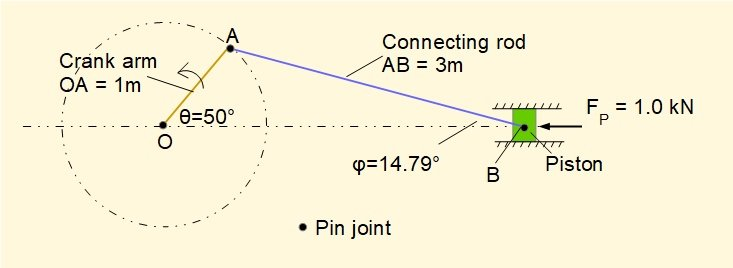Drawing the diagrams

We draw free body diagrams for the three separate elements: piston, connecting rod and crank arm.  The line of action of forces is taken through the centre of the pin joints.  In this case we consider the pins simply as a means of transmitting forces between elements but they could be treated as individual elements with their own diagrams.  The length of an arrow has no significance as regards magnitude of the force.

Free body diagrams for each element are shown below.  The description which follows explains how the diagrams are drawn.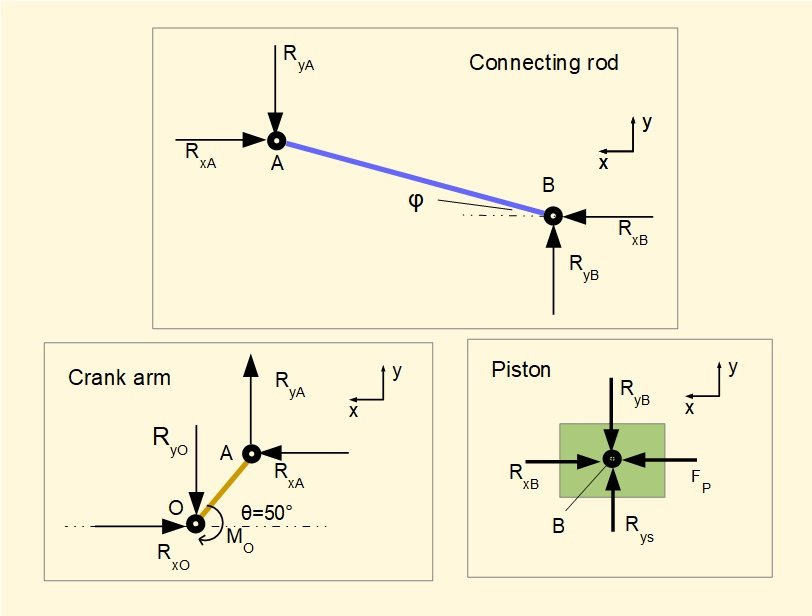Starting with pin B linking the piston and connecting rod, we know the direction and magnitude of FP.  The only other force acting on pin B is from the connecting rod with direction defined by angle φ.  It is convenient to consider forces as components on x and y co-ordinates thus we designate forces from the connecting rod acting on the piston as RxB and RyB.  In this instance directions of RxB and RxY can be assigned intuitively (the connecting rod exerts a horizontal force in a direction opposed to FP and a vertical force downward).

It follows that RyB is accompanied by an equal and opposite force RyS which is the reaction force applied from the cylinder wall to the piston. (We could continue by showing action and reaction forces between the cylinder mountings and ground but this is superfluous for our purpose).  It is not always be possible to draw the direction of a force using intuition, but as long as the given direction is applied in accordance with the co-ordinate axes, signs of calculated forces and moments will ultimately be correct.

Because all forces on the piston act through a common point at pin B there are no moments to consider .

Moving to the diagram for the connecting rod, forces acting on the rod at pin B are equal and opposite to forces RxB and RyB acting on the piston.  At pin A there are forces paired with the crank arm, designated RxA and RyA.  As there are no other forces acting on the connecting rod, the directions of RxA and RyA  must be opposed to RxB and RyB from the equilibrium conditions for forces in x and y directions.

The forces acting on the connecting rod are not directed through a single point hence moments must be considered, either moments of forces at pin A around pin B or vice versa, the choice is arbitrary (see below).

On the crank arm there are forces RxA and RyA at pin A equal and opposite to the forces on the connecting rod.  At pin O there are forces RxO and RyO  transmitted through crankshaft pin O.  In engineering terms pin O is the crankshaft bearing journal.  Because there are no other forces on the crank arm, directions of RxO and RyO are opposed to RxA and RyA.

RxA and RyA both generate a counter-clockwise moment about pin O.  For the crank arm to be in static equilibrium a clockwise moment MO must be applied.

Equilibrium equations and calculations

It is good practice to place all terms initially on the LHS of the equations according  to their sign as per the defined x and y axes.  This ensures any wrongly assigned direction is highlighted when resolving the equations.

Piston

For Σ horizontal forces = 0 :

FP - RxB = 0    gives RxB = FP = 1 kN  --------- (1)

For Σ vertical forces = 0 :

RyS - RyB = 0    gives    RyB = RyS    (the value of RyB is derived from equilibrium conditions for the connecting rod)

Connecting rod

For Σ horizontal forces = 0 :

RxB - RxA = 0    gives    RxB = RxA         gives from (1)    RxA = 1 kN  -------- (2)

For Σ vertical forces = 0 :

RyB - RyA    gives    RyB = RyA -------- (3)

For Σ moments = 0 :

We choose moments of forces RxA and RyA around pin B (counter-clockwise moments are +ve).  RxB and RyB have no moment as they act through B.

From the geometry of the main diagram:     RyA x AB.Cosφ -  RxA x AB.Sinφ = 0         gives    RxA x AB.Sinφ = RyA x AB.Cosφ

gives RyA = RxA x Tanφ    gives from (2)    RyA = 1000 x Tan(14.79°)

gives RyA = 264 N --------(4)   which from (3) gives RyB = RyS = 264 N

Crank arm

For Σ horizontal forces = 0 :

RxA - RxO = 0    gives RxA = RxO    from (2) gives RxA = RxO = 1 kN

For Σ vertical forces = 0 :

RyA - RyO = 0    gives    RyA = RyO     from (4) gives RyA = RyO = 264 N

For Σ moments = 0 :

Moments about O:      RxA x OA.Sinθ + RyA x OA.Cosθ   - MO = 0

gives    MO =  RxA x OA.Sinθ + RyA x OA.Cosθ = 1000 x 1 x Sin(50°) + 264 x 1 x Cos(50°)

gives    MO = 935 Nm

Calculated values for forces and moment are indicated on the diagrams below.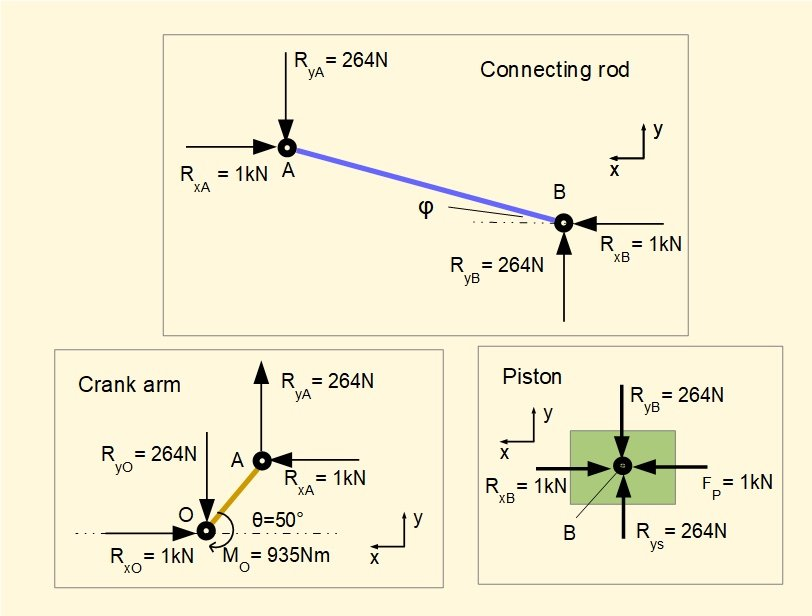The following can be deduced from the diagrams.

• At inner dead centre and outer dead centre positions (i.e. crank angles θ = 0° and 180°) all vertical forces at the pin joints are zero and action of all horizontal forces is through crankshaft pin O.  Thus there is no moment around pin O. At these crank angles an engine produces zero torque.
• For a double-acting engine where the inward stroke is also a power stroke (crank angles from 180° to 360°) the direction of FP acting on the piston is reversed and consequently all horizontal forces on pin joints reverse.  Vertical forces at pin joints do not reverse.  To visualise this imagine the connecting rod pushing down on the piston on the outward stroke and pulling the piston down on the inward stroke.  On both strokes the piston exerts a downward force on the cylinder wall.
• Forces transmitted along the crank arm and connecting rod can be resolved from the components of horizontal and vertical forces along the respective lines as illustrated below.  Forces are considered at pin joint B for the connecting rod and pin joint A for the crank arm.  Pin joints A and O respectively could equally well be used.  In this instance forces have been drawn to scale.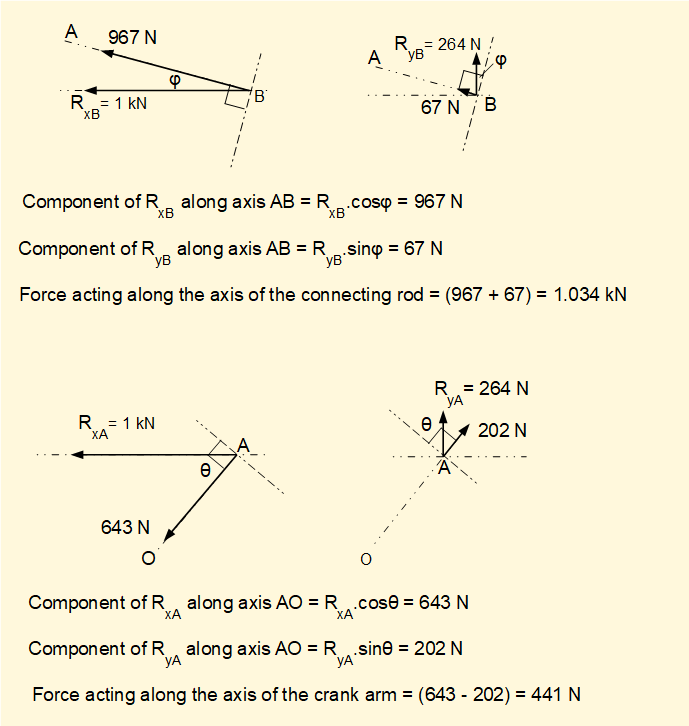#### Example 2     inward compression stroke

The figure below shows the configuration for the inward compression stroke which could apply to a reciprocating gas compressor, the compression stroke of an internal combustion engine or a positive displacement pump.  The crank angle in this example is 310°.  This angle is the mirror image of the crank angle of the engine considered above on its outward stroke.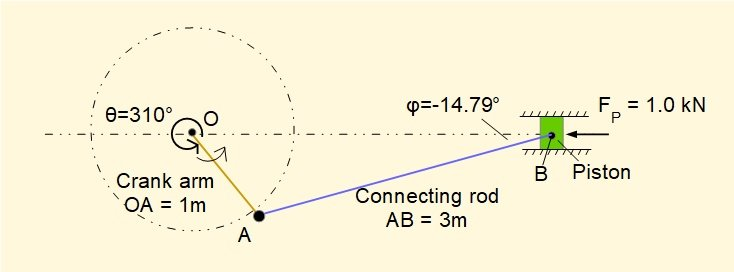The free body diagrams are shown below.  Intuitively we visualise the connecting rod pushing upwards on the piston with the driving torque applied from the crankshaft, resulting in an upward force on the cylinder wall.  Note that the "balancing" torque at crankshaft pin O required for static equilibrium is counter-clockwise.Reversing the direction of rotation* of the crankshaft does not change the direction of forces in the two sets of diagrams above.  The power and compression strokes would now occur when the crank arm is respectively below and above the horizontal centre line between the piston and the crankshaft pin.

* In practice the direction of rotation of internal combustion engines is not reversible being determined by the fixed arrangement of fuel intake and exhaust valves.  Steam engines can be reversed by adjusting the timing of steam inlet and exhaust valves by independent movement of the valve gear.

In the next tutorial on the kinetics of crank mechanisms we examine the effects of inertia forces and moments and the calculation of crankshaft torque.

I welcome feedback at:

### Tutorials - mechanical vibrations

###### Forced vibrations with damping

Alistair's tutorials 2021